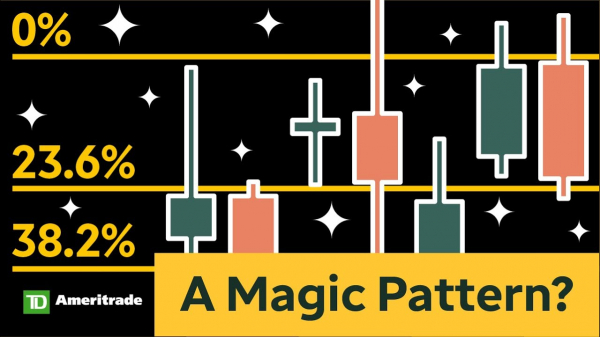# Fibonacci Retracements: A Golden (Ratio) Idea for Trading?#### Key Takeaways

Learn how to create Fibonacci retracement levels on stock charts

Fibonacci retracement levels may be used to identify support and resistance levels

Understand how Fibonacci levels can help make trading decisions

Leonardo Fibonacci was a mathematician who worked on the “golden ratio” and developed the Fibonacci sequence. Many technical traders use what’s known as a Fibonacci retracement to identify support and resistance price levels.

## What is the Fibonacci sequence?

The ” Fibonacci sequence” represents a series of numbers (beginning with 1) in which a given number is equal to the sum of the two preceding numbers:

1, 1, 2, 3, 5, 8, 13, 21, 34, 55, 89, 144, 233, 377, etc.

Fibonacci found that creating squares using the numbers in the sequence for width results in a spiral. The sequence of numbers is ultimately less significant than the ratio from dividing any adjacent number into the other—for example, 8/13 = 0.615. As you move up the sequence, say 34/55, the ratio approaches the number 0.618 or 61.8%. This is known as the golden ratio.

## How Fibonacci applies to trading

In the 1970s, some investors theorized that buying and selling in the stock market might follow patterns similar to those of a natural ecosystem. They began applying Fibonacci numbers to their charts in the form of Fibonacci retracements.

Fibonacci retracements are designed to locate areas of support and resistance on a price chart based on numbers from the golden ratio converted into percentages. The levels include 23.6%, 38.2%, 50%, 61.8%, and 78.6%.

The 38.2% comes from dividing a number in the series by the number found two places to the right, and 23.6% comes from dividing a number by the number found three places to the right. The 50% level isn’t really a Fibonacci number, but many traders still consider it a significant level.

To view these Fibonacci retracement levels, head over to the Charts tab on the thinkorswim® trading platform. After pulling up a chart, select the time frame to analyze and then identify a high or low point. Select Drawings > Drawing Tools % (Fibonacci Retracements). Then place the cursor on the high or low point, click once, move to the next high or low point to the right, and click again. The tool automatically calculates the corresponding Fibonacci levels based on percentage retracements (see figure 1).FIGURE 1: RANGE OF FIBONACCI RETRACEMENT LEVELS. By plotting the lowest and highest points on this chart, the Fibonacci retracement tool automatically calculates potential resistance levels following a major low. Chart source: thinkorswim platform. For illustrative purposes only. Past performance does not guarantee future results.

## How to use Fibonacci retracements

In the chart of the S&P 500 index (SPX) in figure 1, notice the top level is 100% and the bottom level is 0%. The retracement levels in between are areas you can watch for potential technical support or resistance levels. Fibonacci retracements can also be used in the opposite way—from a low point to a high point (as long as the high point is to the right of the low one). In this scenario, retracing a move higher, the Fibonacci series frequently acts as key points of support in the pullback or correction.

When the SPX started to move above its March low, it met slight resistance at the 23.6% level. Even though it broke above it, the close was right around that level. The SPX then continued moving toward the 38.2% level, hesitated there for a few days, and then went back toward the 23.6% level. When reviewing the price chart, it’s possible to see how the different retracement levels acted as support and resistance levels.

Traders can try applying this tool to individual stocks. It’s also possible to combine Fibonacci levels with other indicators to get more trading signals.

Fibonacci retracements are accepted and used by many traders, including some who trade for large institutions and hedge funds. There’s no guarantee that using Fibonacci retracements will work effectively as part of a trading strategy, but they can provide some levels to watch when engaging in technical analysis.

After becoming familiar with the Fibonacci sequence and understanding how to use retracements in trading, traders can also explore some lesser-known technical tools to add to their trading toolbox.

### Amp up your investing IQ.Fibonacci Numbers